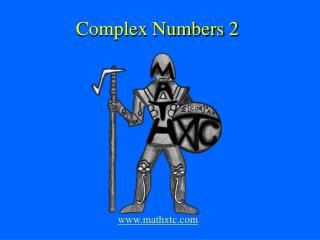Download PresentationComplex Numbers 2

# Complex Numbers 2 - PowerPoint PPT PresentationDownload Presentation## Complex Numbers 2

- - - - - - - - - - - - - - - - - - - - - - - - - - - E N D - - - - - - - - - - - - - - - - - - - - - - - - - - -
##### Presentation Transcript

1. Complex Numbers 2 www.mathxtc.com

2. Complex Numbers

3. Complex NumbersWhat is truth?

4. Complex NumbersWho uses themin real life?

5. Complex NumbersWho uses themin real life?Here’s a hint….

6. Complex NumbersWho uses themin real life?Here’s a hint….

7. Complex NumbersWho uses themin real life? The navigation system in the space shuttle depends on complex numbers!

8. -2 Can you see a problem here?

9. -2 Who goes first?

10. -2 Complex numbers do not have order

11. What is a complex number? • It is a tool to solve an equation.

12. What is a complex number? • It is a tool to solve an equation. • It has been used to solve equations for the last 200 years or so.

13. What is a complex number? • It is a tool to solve an equation. • It has been used to solve equations for the last 200 years or so. • It is defined to be isuch that ;

14. What is a complex number? • It is a tool to solve an equation. • It has been used to solve equations for the last 200 years or so. • It is defined to be isuch that ; • Or in other words;

15. Complex • i is an imaginary number

16. Complex • i is an imaginary number • Or a complex number

17. Complex • i is an imaginary number • Or a complex number • Or an unreal number

18. Complex? • i is an imaginary number • Or a complex number • Or an unreal number • The terms are inter-changeable unreal complex imaginary

19. Some observations • In the beginning there were counting numbers 1 2

20. Some observations • In the beginning there were counting numbers • And then we needed integers 1 2

21. Some observations • In the beginning there were counting numbers • And then we needed integers 1 2 -1 -3

22. Some observations • In the beginning there were counting numbers • And then we needed integers • And rationals 1 0.41 2 -1 -3

23. Some observations • In the beginning there were counting numbers • And then we needed integers • And rationals • And irrationals 1 0.41 2 -1 -3

24. Some observations • In the beginning there were counting numbers • And then we needed integers • And rationals • And irrationals • And reals 1 0.41 2 -1 0 -3

25. So where do unreals fit in ? We have always used them. 6 is not just 6 it is 6 + 0i. Complex numbers incorporate all numbers. 3 + 4i 2i 1 0.41 2 -1 0 -3

26. A number such as 3i is a purely imaginary number

27. A number such as 3i is a purely imaginary number • A number such as 6 is a purely real number

28. A number such as 3i is a purely imaginary number • A number such as 6 is a purely real number • 6 + 3i is a complex number

29. A number such as 3i is a purely imaginary number • A number such as 6 is a purely real number • 6 + 3i is a complex number • x + iy is the general form of a complex number

30. A number such as 3i is a purely imaginary number • A number such as 6 is a purely real number • 6 + 3i is a complex number • x + iy is the general form of a complex number • If x + iy = 6 – 4i then x = 6 and y = -4

31. A number such as 3i is a purely imaginary number • A number such as 6 is a purely real number • 6 + 3i is a complex number • x + iy is the general form of a complex number • If x + iy = 6 – 4i then x = 6 and y = –4 • The ‘real part’ of 6 – 4i is 6

32. Worked Examples • Simplify

33. Worked Examples • Simplify

34. Worked Examples • Simplify • Evaluate

35. Worked Examples • Simplify • Evaluate

36. Worked Examples 3. Simplify

37. Worked Examples 3. Simplify

38. Worked Examples 3. Simplify 4. Simplify

39. Worked Examples 3. Simplify 4. Simplify

40. Worked Examples 3. Simplify 4. Simplify 5. Simplify

41. Addition Subtraction Multiplication 3. Simplify 4. Simplify 5. Simplify

42. Division 6. Simplify

43. Division 6. Simplify The trick is to make the denominator real:

44. Division 6. Simplify The trick is to make the denominator real: